Custom SearchOther Applications The applications of powers of 10 may be broadened to include problems involving reciprocals and powers of products. RECIPROCALS.-The following example illustrates the use of powers of 10 in the formation of a reciprocal: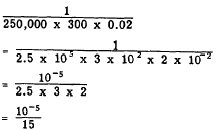Rather than write the numerator as 0.00001, write it as the product of two factors, one of which may be easily divided, as follows: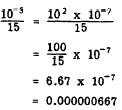POWER OF A PRODUCT.-The following example illustrates the use of powers of 10 in finding the power of a product: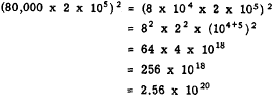RADICALS An expression such as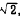,, orthat exhibits a radical sign, is referred to as a RADICAL. We have already worked with radicals in the form of fractional exponents, but it is also frequently necessary to work with them in the radical form. The word "radical" is derived from the Latin word "radix," which means "root." The word "radix" itself is more often used in modern mathematics to refer to the base of a number system, such as the base 2 in the binary system. However, the word "radical" is retained with its original meaning of "root." The radical symbol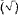appears to be a distortion of the initial letter "r" from the word "radix." With long usage, the r gradually lost its significance as a letter and became distorted into the symbol as we use it. The vinculum helps to specify exactly which of the letters and numbers following the radical sign actually belong to the radical expression. The number under a radical sign is the RADICAND. The index of the root (except in the case of a square root) appears in the trough of the radical sign. The index tells what root of the radicand is intended. For example, in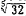, the radicand is 32 and the index of the root is 5. The fifth root of 32 is intended. In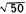, the square root of 50 is intended. When the index is 2, it is not written, but is understood. If we can find one square root of a number we can always find two of them. Remember (3)2 is 9 and (-3)2 is also 9. Likewise (4)2 and (-4)2 both equal 16 and (5)2 and (-5)2 both equal 25. Conversely,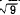is +3 or -3,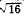is +4 or -4, andis +5 or -5. When we wish to show a number that may be either positive or negative, we may use the symbol + which is read "plus or minus." Thus 3 means "plus or minus 3." Usually when a number is placed under the radical sign, only its positive root is desired and, unless otherwise specified, it is the only root that need be found. COMBINING RADICALS A number written in front of another number and intended as a multiplier is called a COEFFICIENT. The expression 5x means 5 times x; means a times y; and 7means 7 times. In these examples, 5 is the coefficient of x, a is the coefficient of y, and 7 is the coefficient of. Radicals having the same index and the same radicand are SIMILAR. Similar radicals may have different coefficients in front of the radical sign. For example, 3,, and 1/5are similar radicals. When a coefficient is not written, it is understood to be 1. Thus, the coefficient ofis 1. The rule for adding radicals is the same as that stated for adding denominate numbers: Add only units of the same kind. For example, we could add 2and 4because the "unit" in each of these numbers is the same (). By the same reasoning, we could not add 2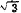and 4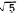because these are not similar radicals. Addition and Subtraction When addition or subtraction of similar radicals is indicated, the radicals are combined by adding or subtracting their coefficients and placing the result in front of the radical. Adding 3and 5is similar to adding 3 bolts and 5 bolts. The following examples illustrate the addition and subtraction of similar radical expressions: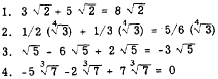Example 4 illustrates a case that is sometimes troublesome. The sum of the coefficients, -5, -2, and 7, is 0. Therefore, the coefficient of the answer would be 0, as follows: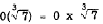Thus the final answer is 0, since 0 multiplied by any quantity is still 0. Practice problems. Perform the indicated operations:Answers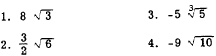Integrated Publishing, Inc. - A (SDVOSB) Service Disabled Veteran Owned Small Business